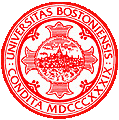## CAS CS 585 Image and Video Computing - Spring 2021

Homework due date: 1 day before exam, which is Wednesday, February 24, 9:30 am, No late submissions accepted because solutions will be immediately published.

Exercise 1: Hausdorff Distance

There are two shapes in the figure below. The triangle in red has three vertices: A(-2,3), B(3,1), and C(0,-3). The rectangle in blue has four vertices: D(-3,2), E(2,2), F(2,-1), and G(-3, -1).1. Consider two point sets S1 and S2, where S1={A, B, C} and S2={D, E, F, G}. Calculate the Hausdorff distance between these two point sets.
2. Now consider all the points forming these two polygons. What's the Hausdorff distance between the triangle and the rectangle?

Exercise 2: Segmentation

The following tables include all the local maxima and local minima of the grayscale histogram of an image.

Table 1: Local Maxima

 Gray Values # of Pixels 52 54 103 231 1000 1170 1750 1300

Table 2: Local Minima

 Gray Values # of Pixels 0 53 75 157 255 500 890 240 190 310

Calculate the highest peakiness and the corresponding threshold using Mode Method.

Programming Assignment on Segmentation

The programming component of this homework will be published after the exam due date.